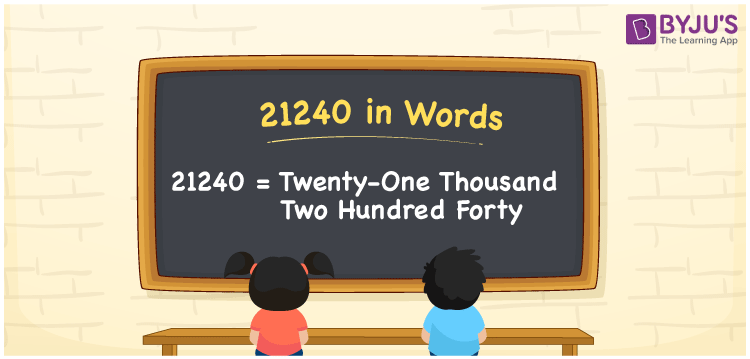# 21240 in Words

21240 in words is written as Twenty-One Thousand Two Hundred Forty. For example, if you bought a new mobile worth Rs. 21240, you can say, “I bought a new mobile worth Rupees Twenty-One Thousand Two Hundred Forty”. Place value chart is very useful in writing the numbers in word form. 21240 is a cardinal number since it represents a certain amount. Let us learn the spelling of 21240 in English in this article.

 21240 in Words Twenty-One Thousand Two Hundred Forty Twenty-One Thousand Two Hundred Forty in numerical form 21240

## 21240 in English Words

We know that numbers in words are written using the English alphabet. Hence, 21240 in English words is read as Twenty-One Thousand Two Hundred Forty.## How to Write 21240 in Words?

Using a place value chart, let us write the number 21240 in words. Since 21240 is a five-digit number, so let’s create a chart of five columns as shown below:

 Ten-Thousands Thousands Hundreds Tens Ones 2 1 2 4 0

Thus, we can write the expanded form as:

2 x Ten Thousand + 1 x Thousand + 2 x Hundred + 4 x Ten + 0 x One

= 2 x 10000 + 1 x 1000 + 2 x 100 + 4 x 10 + 0 x 1

= 20000 + 1000 + 200 + 40 + 0

= 20000 + 1000 + 200 + 40

= 21240

= Twenty-One Thousand Two Hundred Forty

Therefore, 21240 in words is written as Twenty-One Thousand Two Hundred Forty

Interesting way of writing 21240 in words

2 = Two

21 = Twenty-One

212 = Two Hundred and Twelve

2124 = Two Thousand One Hundred Twenty-Four

21240 = Twenty-One Thousand Two Hundred Forty

Thus, the word form of the number 21240 is Twenty-One Thousand Two Hundred Forty

21240 is a natural number that is the successor of 21239 and the predecessor of 21241

• 21240 in words – Twenty-One Thousand Two Hundred Forty
• Is 21240 an odd number? – No
• Is 21240 an even number? – Yes
• Is 21240 a perfect square number? – No
• Is 21240 a perfect cube number? – No
• Is 21240 a prime number? – No
• Is 21240 a composite number? – Yes

## Frequently Asked Questions on 21240 in Words

Q1

### Write 21240 in words.

21240 in words is written as Twenty-One Thousand Two Hundred Forty.
Q2

### What is the place value of 1 in 21240?

The place value of 1 in 21240 is Thousands.
Q3

### 21240 is a prime number. True or False.

False, 21240 is not a prime number.